# AP Statistics : How to establish an alternate hypothesis

## Example Questions

### Example Question #3 : Significance Logic And Establishing Hypotheses

A researcher stated thatpercent of Americans in the 2008 presidential election were liberal. The researcher wants to find evidence that the percent of liberal Americans was different for the 2012 presidential election. Which of the hypotheses best describes the question that the researcher is trying to answer?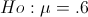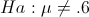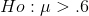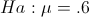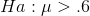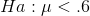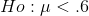Explanation:

The question states that the researcher thinks the percent of liberal Americans in 2012 is different than thepercent in 2008. The hypothesis is 2 directional. Therefore the null hypothesis is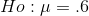and the alternative is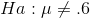.

### Example Question #4 : Significance Logic And Establishing Hypotheses

The department of labor reports that umemployment is down to 5% in the United States.  A politician claims that the data was manipulated to show better numbers. The politician believes our country is worse off that unemployment must be worse than the claim made by the department of labor.  What is the alternative hypothesis for a test for this situation?

Ha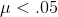Ho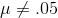Ho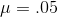Ha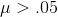HaHaExplanation:

The alternative hypthesis is denoted by Ha. The alternative represents the value that is being tested for and not the original claim.  The original claim for the problem is unemployment is%.  The test claim is that it is greater than%.  This means the alternative must be.

### Example Question #5 : Significance Logic And Establishing Hypotheses

A researcher wants to determine whether there is a significant linear relationship between time spent meditating and time spent studying. What is the appropriate alternative hypothesis for this study?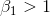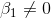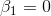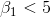Explanation:

This question is about a linear regression between time spent meditating and time spent studying. Therefore, the hypothesis is regarding Beta1, the slope of the line. We are testing a non-directional or bi-directional claim that the relationship is significant. A slope of zero indicates no relationship between the two variables. Therefore, the alternative hypothesis is that the slope is not equal to zero.

### Example Question #6 : Significance Logic And Establishing Hypotheses

A researcher wants to compare 3 different treatments to see if any of the treatments affects study time. The three treatments studied are control group, a group given vitamins, and a group given a placebo.  They found that the average time spent studying with control students was 2 hours, with students given vitamins it was 3 hours, and with placebos students studied 5 hours. Which of the following is the correct alternative hypothesis?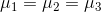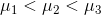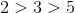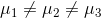Not all of the means are equal

Not all of the means are equal

Explanation:

Because we are comparing more than 2 groups, we must use an ANOVA for this problem. For an ANOVA problem, the null hypothesis is that not all of the groups’ means are the same.is not correct because this hypothesis implies that all of the groups are different, which is not actually tested by the basic ANOVA test.

### Example Question #1 : How To Establish An Alternate Hypothesis

A researcher wants to investigate the claim that taking vitamins will help a student study longer. First, the researcher collects 32 students who do not take vitamins and determines their time spent studying. Then, the 32 students are given a vitamin for 1 week. After 1 week of taking vitamins, students are again tested to determine their time spent studying. Which of the following is the correct alternative hypothesis?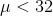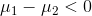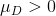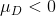Explanation:

Because the same students are tested twice, this is a paired study, therefore we must use a hypothesis appropriate for a paired t-test.  The hypothesis for a paired t-test regards the average of the differences between before and after treatment, called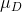. We are testing the claim that vitamins increase study time, therefore this will be the alternative hypothesis. This means that the difference between control – treatment is greater than zero, since the average study time for vitamin users would be greater than that of the control.

### Example Question #2 : How To Establish An Alternate Hypothesis

A study would like to determine whether meditation helps students improve focus time. They know that the average focus time of an American 4th grader is 23 minutes. They then recruit 50 meditators and calculate their average focus time. What is the appropriate alternative hypothesis for this study?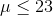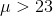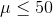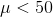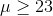Explanation:

Because we are comparing one sample to a known mean, the hypothesis takes the form of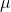compared to the known value.  We would like to test the claim that meditating will increase focus time, so this will be the alternative hypothesis. Therefore, the alternative thatis greater than the average of 23 minutes.

### Example Question #31 : Inference

A study would like to determine whether meditation helps students increase focus time. They used a control group of 30 students and compared their focus time to a group of 30 meditating students and compared their average time spent meditating.  What is the appropriate alternative hypothesis for this study?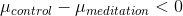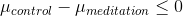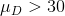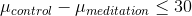Because we are comparing two samples, the hypothesis takes the form of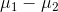. The study is testing the claim that meditation helps increase focus time, so this will be our alternative hypothesis. Our alternative hypothesis in words is that the difference between control and meditation groups is less than zero. That is because we think average time for meditators will be greater than the control group’s average.